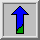# Erosion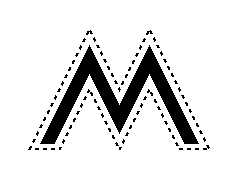Common Names: Erode, Shrink, Reduce

## Brief Description

Erosion is one of the two basic operators in the area of mathematical morphology, the other being dilation. It is typically applied to binary images, but there are versions that work on grayscale images. The basic effect of the operator on a binary image is to erode away the boundaries of regions of foreground pixels (i.e. white pixels, typically). Thus areas of foreground pixels shrink in size, and holes within those areas become larger.

## How It Works

Useful background to this description is given in the mathematical morphology section of the Glossary.

The erosion operator takes two pieces of data as inputs. The first is the image which is to be eroded. The second is a (usually small) set of coordinate points known as a structuring element (also known as a kernel). It is this structuring element that determines the precise effect of the erosion on the input image.

The mathematical definition of erosion for binary images is as follows:

Suppose that X is the set of Euclidean coordinates corresponding to the input binary image, and that K is the set of coordinates for the structuring element.

Let Kx denote the translation of K so that its origin is at x.

Then the erosion of X by K is simply the set of all points x such that Kx is a subset of X.

The mathematical definition for grayscale erosion is identical except in the way in which the set of coordinates associated with the input image is derived. In addition, these coordinates are 3-D rather than 2-D.

As an example of binary erosion, suppose that the structuring element is a 3×3 square, with the origin at its center as shown in Figure 1. Note that in this and subsequent diagrams, foreground pixels are represented by 1's and background pixels by 0's.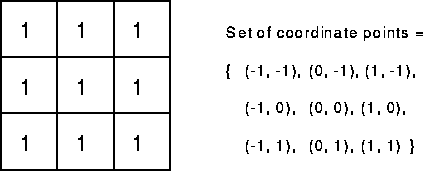Figure 1 A 3×3 square structuring element

To compute the erosion of a binary input image by this structuring element, we consider each of the foreground pixels in the input image in turn. For each foreground pixel (which we will call the input pixel) we superimpose the structuring element on top of the input image so that the origin of the structuring element coincides with the input pixel coordinates. If for every pixel in the structuring element, the corresponding pixel in the image underneath is a foreground pixel, then the input pixel is left as it is. If any of the corresponding pixels in the image are background, however, the input pixel is also set to background value.

For our example 3×3 structuring element, the effect of this operation is to remove any foreground pixel that is not completely surrounded by other white pixels (assuming 8-connectedness). Such pixels must lie at the edges of white regions, and so the practical upshot is that foreground regions shrink (and holes inside a region grow).

Erosion is the dual of dilation, i.e. eroding foreground pixels is equivalent to dilating the background pixels.## Guidelines for Use

Most implementations of this operator will expect the input image to be binary, usually with foreground pixels at intensity value 255, and background pixels at intensity value 0. Such an image can often be produced from a grayscale image using thresholding. It is important to check that the polarity of the input image is set up correctly for the erosion implementation being used.

The structuring element may have to be supplied as a small binary image, or in a special matrix format, or it may simply be hardwired into the implementation, and not require specifying at all. In this latter case, a 3×3 square structuring element is normally assumed which gives the shrinking effect described above. The effect of an erosion using this structuring element on a binary image is shown in Figure 2.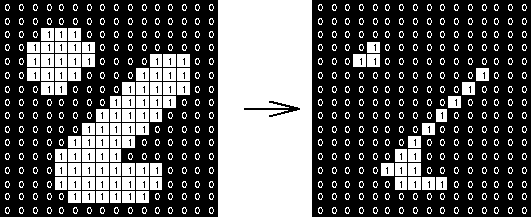Figure 2 Effect of erosion using a 3×3 square structuring element

The 3×3 square is probably the most common structuring element used in erosion operations, but others can be used. A larger structuring element produces a more extreme erosion effect, although usually very similar effects can be achieved by repeated erosions using a smaller similarly shaped structuring element. With larger structuring elements, it is quite common to use an approximately disk shaped structuring element, as opposed to a square one.

The imageis the result of eroding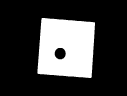four times with a disk shaped structuring element 11 pixels in diameter. It shows that the hole in the middle of the image increases in size as the border shrinks. Note that the shape of the region has been quite well preserved due to the use of a disk shaped structuring element. In general, erosion using a disk shaped structuring element will tend to round concave boundaries, but will preserve the shape of convex boundaries.

Erosions can be made directional by using less symmetrical structuring elements. For example, a structuring element that is 10 pixels wide and 1 pixel high will erode in a horizontal direction only. Similarly, a 3×3 square structuring element with the origin in the middle of the top row rather than the center, will erode the bottom of a region more severely than the top.

Grayscale erosion with a flat disk shaped structuring element will generally darken the image. Bright regions surrounded by dark regions shrink in size, and dark regions surrounded by bright regions grow in size. Small bright spots in images will disappear as they are eroded away down to the surrounding intensity value, and small dark spots will become larger spots. The effect is most marked at places in the image where the intensity changes rapidly, and regions of fairly uniform intensity will be left more or less unchanged except at their edges. Figure 3 shows a vertical cross-section through a graylevel image and the effect of erosion using a disk shaped structuring element. Note that the flat disk shaped kernel causes small peaks in the image to disappear and valleys to become wider.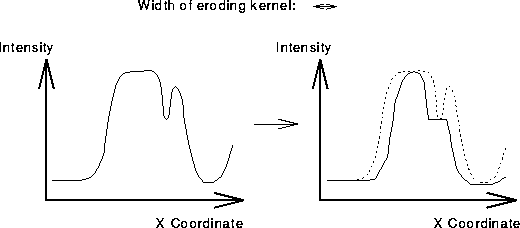Figure 3 Graylevel erosion using a disk shaped structuring element. The graphs show a vertical cross-section through a graylevel image.

The image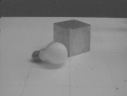illustrates graylevel erosion. It was produced fromby two erosion passes using a 3×3 flat square structuring element. Note that the highlights have disappeared, and that many of the surfaces seem more uniform in appearance due to the elimination of bright spots. The body of the cube has grown in size since it is darker than its surroundings. The effect of five passes of the same erosion operator on the original image is shown in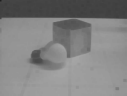There are many specialist uses for erosion. One of the more common is to separate touching objects in a binary image so that they can be counted using a labeling algorithm. The image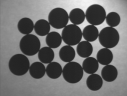shows a number of dark disks (coins in fact) silhouetted against a light background. The result of thresholding the image at pixel value 90 yields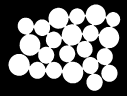It is required to count the coins. However, this is not going to be easy since the touching coins form a single fused region of white, and a counting algorithm would have to first segment this region into separate coins before counting, a non-trivial task. The situation can be much simplified by eroding the image. The imageshows the result of eroding twice using a disk shaped structuring element 11 pixels in diameter. All the coins have been separated neatly and the original shape of the coins has been largely preserved. At this stage a labeling algorithm can be used to count the coins. The relative sizes of the coins can be used to distinguish the various types by, for example, measuring the area of each distinct region.

The imageis derived from the same input picture, but a 9×9 square structuring element is used instead of a disk (the two structuring elements have approximately the same area). The coins have been clearly separated as before, but the square structuring element has led to distortion of the shapes, which is some situations could cause problems in identifying the regions after erosion.

Erosion can also be used to remove small spurious bright spots (`salt noise') in images. The imageshows an image with salt noise, andshows the result of erosion with a 3×3 square structuring element. Note that although the noise has been removed, the rest of the image has been degraded significantly. Compare this with the same task using opening.

We can also use erosion for edge detection by taking the erosion of an image and then subtracting it away from the original image, thus highlighting just those pixels at the edges of objects that were removed by the erosion. An example of a very similar technique is given in the section dealing with dilation.

Finally, erosion is also used as the basis for many other mathematical morphology operators.

## Interactive Experimentation

You can interactively experiment with this operator by clicking here.

## Exercises

1. What would be the effect of an erosion using the cross-shaped structuring element shown in Figure 4?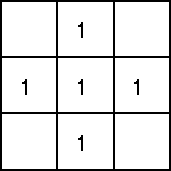Figure 4 Cross-shaped structuring element

2. Is there any difference in the final result between applying a 3×3 square structuring element twice to an image, and applying a 5×5 square structuring element just once to the image? Which do you think would be faster and why?

3. When using large structuring elements, why does a disk shaped structuring element tend to preserve the shapes of convex objects better than a square structuring element?

4. Use erosion in the way described above to detect the edges ofIs the result different to the one obtained with dilation?

## References

R. Gonzalez and R. Woods Digital Image Processing, Addison-Wesley Publishing Company, 1992, pp 518, 512, 550.

R. Haralick and L. Shapiro Computer and Robot Vision, Vol. 1, Chap. 5, Addison-Wesley Publishing Company, 1992.

A. Jain Fundamentals of Digital Image Processing, Prentice-Hall, 1986, p 384.

D. Vernon Machine Vision, Prentice-Hall, 1991, pp 63 - 66, 76 - 78.

## Local Information